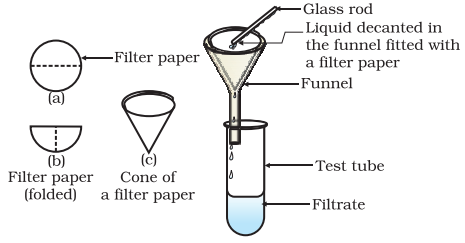Share

# Solution - Concentration of a Solution

#### notes

In activity 2.2, we observed that groups A and B obtained different shades of solutions. So, we understand that in a  solution the relativeproportion of the solute and solvent can be varied. Depending upon the amount of solute present in a solution, it can be called a dilute, concentrated or a saturated solution. Dilute  and concentrated are comparative terms. In activity 2.2, the solution obtained by group A is dilute as compared to that obtained by group B proportion of the solute and solvent can be varied. Depending upon the amount of solute present in a solution, it can be called a dilute, concentrated or a saturated solution. Dilute and concentrated are comparative terms. In activity 2.2, the solution obtained by group A is dilute as compared to that obtained by group B.1. Activity :

• Take approximately 50 mL of water each in two separate beakers.
• Add salt in one beaker and sugar or barium chloride in the second beaker with continuous stirring.
• When no more solute can be dissolved, heat the contents of the beaker to raise the temperature by about 5°C.
• Start adding the solute again.

Is the amount of salt and sugar or barium chloride, that can be dissolved in water at a given temperature, the same?
At any particular temperature, a solution that has dissolved as much solute as it is capable of dissolving, is said to be a saturated solution. In other words, when no more solute can be dissolved in a solution at a given temperature, it is called a saturated solution. The amount of the solute present in the saturated solution at this temperature is called its solubility.

1. Example  :

A solution contains 40 g of common salt in 320 g of water. Calculate the concentration in terms of mass by mass percentage of the solution.

Solution:

Mass of solute (salt) = 40 g
Mass of solvent (water) = 320 g
We know,
Mass of solution = Mass of solute + Mass of solvent
= 40 g + 320 g
= 360 g
Mass percentage of solution ="Mass of solute"/"Mass of solution"xx100
40/360xx100=11.1%

S